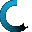Retrieving data ...
Please do not click anywhere else.

If you see no response after 2-3 minutes, then click on the [X] button in the upper right corner to close the window and retry the operation at a later time.
Interactive Multimedia Learning & Teaching Management System Suite## Course Contents

Course Name: Algebra 1 (8th/9th Grade)
• 1. The Basics
•  1.1 Understanding Numbers
•  1.1.1 Whole Numbers
•  1.1.2 Positive and Negative Numbers
•  1.1.3 Integers
•  1.1.4 Decimals
•  1.1.5 Real Numbers
•  1.1.6 Prime Numbers
•  1.1.7 Rational & Irrational Numbers
•  1.1.8 Percents
•  1.2 Fractions
•  1.2.1 Defining Fractions
•  1.2.2 Reducing Fractions
•  1.2.3 Adding Fractions
•  1.2.4 Adding Fractions and whole numbers
•  1.2.5 Multiplying fractions
•  1.2.6 Multiplying fractions and whole numbers
•  1.2.7 Subtracting Fractions
•  1.2.8 Subtracting Fractions and whole numbers
•  1.2.9 Dividing Fractions
•  1.2.10 Dividing Fractions and whole numbers
•  1.2.11 Mixed Fractions Operations
•  1.3 Basic Real Number Operations
•  1.3.1 Number Comparisons
•  1.3.2 Properties of Real Numbers
•  1.3.4 Subtractions
•  1.3.5 Multiplication
•  1.3.6 Division
•  1.3.7 Order of Operation
•  1.3.8 Mixed Operations
•  1.4 Exponents
•  1.4.1 Understanding Exponents
•  1.4.2 Multiplying Exponents with the Same Base
•  1.4.3 Multiplying Fractional Exponents with the Same Base
•  1.4.4 Multiplying Exponents Raised to an Exponent
•  1.4.5 Expressions Raised to a Power
•  1.4.6 Fractions Raised to a Power
•  1.4.7 More Multiplying Exponents
•  1.4.8 Negative Exponents
•  1.4.9 Multiplying with Negative Exponents
•  1.4.10 Dividing with Exponents
•  1.5 Rates and Dimensional Analysis
• 2. Introduction to Variables and Algebraic Expressions
•  2.1 Defining Variable
•  2.2 Basic Operations with Variables
•  2.2.1 Additions and Subtractions
•  2.2.2 Multiplications
•  2.2.3 Divisions
•  2.2.4 Mixed Operations with Variables
•  2.3 Fractions with Variables
•  2.4 Canceling with Variable
•  2.5 Simplifying and Evaluating Expressions
•  2.6 Combining Like Terms
•  2.7 Word Problems
• 3. Solving Linear Equations
•  3.1 Writing Equations
•  3.2 Solving Equations Using Addition and Subtraction
•  3.3 Solving Equations Using Multiplication and Division
•  3.4 Solving Multi-step Equations
•  3.5 Solving Equations with the Variable on each side
•  3.6 Ratios and Proportions
•  3.7 Solving Equations and Formulas
• 4. Graphing Relations and Functions
•  4.1 The Number Line and the Coordinate Plane
•  4.2 Transformations on the Coordinate Plane
•  4.3 Relations
•  4.4 Equations as Relations
•  4.5 Graphing Linear Equations
•  4.6 Functions
•  4.7 Arithmetic Sequences
•  4.8 Writing Equations from Patterns
•  4.9 Interpreting a Graph Representing a Given Situation
•  4.10 Sketching a Graph Representing a Given Situation
• 5. Linear Equations
•  5.1 Slope
•  5.2 Slope-Intercept Form
•  5.3 Writing Equations in Slope-Intercept Form
•  5.4 Writing Equations Using Point and Slope
•  5.5 Writing Equations in a Point-Slope Form
•  5.6 Graphing Linear Equations Using Slope/Intercept Method
•  5.7 Writing Parallel Equations
•  5.8 Writing Perpendicular Equations
• 6. Solving Linear Inequalities
•  6.1 Linear Inequalities
•  6.2 Solving Linear Inequalities by Addition and Subtraction
•  6.3 Solving Linear Inequalities by Multiplication and Division
•  6.4 Solving Multi-step Inequalities
•  6.5 Solving Compound Inequalities
•  6.6 Solving Open Sentences Involving Absolute Value
•  6.7 Graphing Inequalities with two Variables
•  6.8 Solving Linear Inequalities Using Solutions Sets
• 7. Solving Systems of Linear Equations and Inequalities
•  7.1 Graphing Systems of Equations
•  7.2 Substitution
•  7.3 Elimination using Addition and Subtraction
•  7.4 Elimination using Multiplication
•  7.5 Graphing System of Inequalities
• 8. Polynomials
•  8.1 Monomials and Degree of Monomials
•  8.2 Multiplying Monomials
•  8.3 Dividing Monomials
•  8.4 Polynomials and Degree of Polynomials
•  8.5 Adding and Subtracting Polynomials
•  8.6 Multiplying a Polynomial by Monomial
•  8.7 Multiplying Polynomials
•  8.8 Special Products of Polynomials
•  8.9 Difference of Squares
•  8.10 Monomial Roots and Powers
•  8.11 Dividing a Polynomial by Monomial
• 9. Factoring
•  9.1 Factors and Greatest Common Factor
•  9.2 Factoring Using the Distributive Property
•  9.3 Factoring Trinomials: x^2 + bx + c
•  9.4 Factoring Trinomials: ax^2 + bx + c
•  9.5 Factoring Difference of Squares
•  9.6 Perfect Squares and Factoring
•  9.7 Solving Quadratic Equations by factoring
•  9.8 Simplifying Algebraic Ratios
•  9.9 Solve Algebraic Proportions
• 10. Quadratic Equations and Functions
•  10.1 Solve Quadratic Equations by the Square Root Method
•  10.2 Solving Quadratic Equations by Completing the Square
•  10.3 Solving Quadratic Equations using the Quadratic Formula
•  10.4 Graphing Quadratic Equations
•  10.5 Solving Quadratic Equations by Graphing
•  10.6 The Discriminant
•  10.7 The Sum and the Product of the Solutions
•  10.8 Solving Quadratic Equations with Perfect Squares
•  10.9 Solving Quadratic Equations That Contain Radical Expressions
•  10.10 Word Problems Using Quadratic Formula
• 11. Radical Expressions and Triangles
•  11.1 Square Roots
•  11.2 Simplifying Radical Expressions
•  11.3 Operations with Radical Expressions
•  11.3.1 Adding and Subtracting Radical Expressions
•  11.3.2 Multiplying Radical Expressions
•  11.3.3 Dividing Radical Expressions
•  11.4 Pythagorean Theorem
•  11.5 The Distance Formula
•  11.6 Similar Triangles
•  11.7 Cube Roots
•  11.8 Rational Exponents
•  11.9 Comparing Real Number Expressions